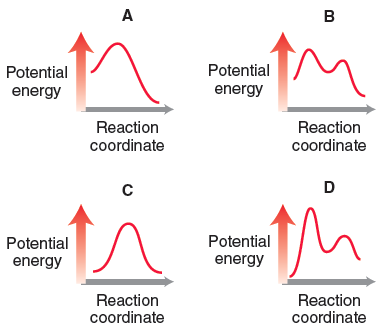# Problem: Consider the following four energy diagrams:Compare all four energy diagrams. Which one exhibits the largest E a?

###### FREE Expert Solution
86% (185 ratings)
###### Problem Details

Consider the following four energy diagrams:

Compare all four energy diagrams. Which one exhibits the largest E a?